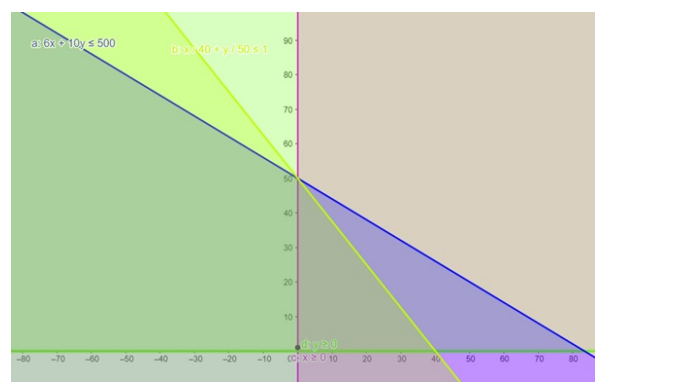# If a young man rides his motorcycle at 40 km per hour,

Question:

If a young man rides his motorcycle at $40 \mathrm{~km}$ per hour, he has to spend $6 \mathrm{per} \mathrm{km}$ on petrol and if he rides it at $50 \mathrm{~km}$ hour, the petrol cost rises to 10 per $\mathrm{km}$. He has 500 to spend on petrol and wishes to find the maximum distance he can travel within one hour. Formulate the data in the form of inequation and draw a graph representing the solution of these inequations.

Solution:

Let the distance covered with speed $40 \mathrm{~km} / \mathrm{hr}=\mathrm{x} \mathrm{km}$

And the distance covered with speed $50 \mathrm{~km} / \mathrm{hr}=\mathrm{y} \mathrm{km}$

We know that,

Time $=\frac{\text { Distance }}{\text { Speed }}$

Therefore, maximum speed covered within one hour is

$\frac{x}{40}+\frac{y}{50} \leq 1$

Thus, according to equation,

Maximum speed covered,$Z_{\max }=x+y$

Subject to the constraint,

$6 x+10 y \leq 500$

$\frac{x}{40}+\frac{y}{50} \leq 1$

$x, y \geq 0$

Now plotting both the line on graph paper, we have,Distance covered with speed $40 \mathrm{~km} / \mathrm{hr}=\mathrm{x}=0$

Distance covered with speed $50 \mathrm{~km} / \mathrm{hr}=\mathrm{y}=50$

Therefore, maximum distance covered $=0+50=50 \mathrm{~km}$